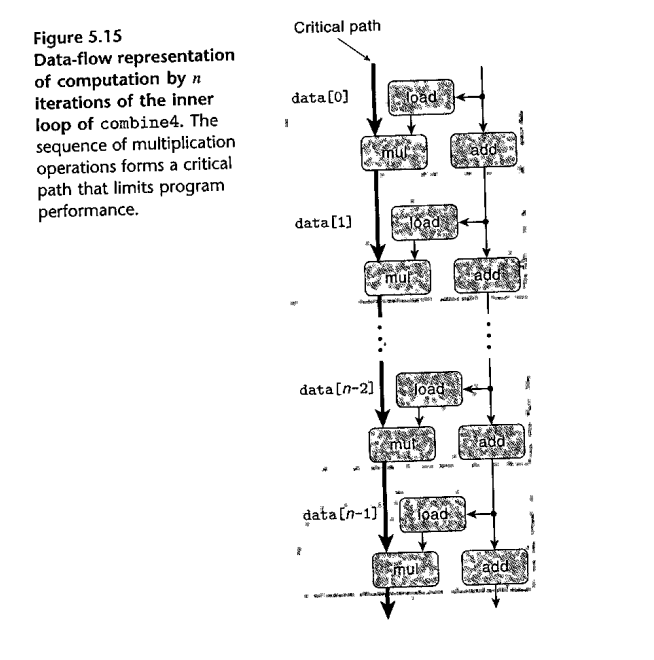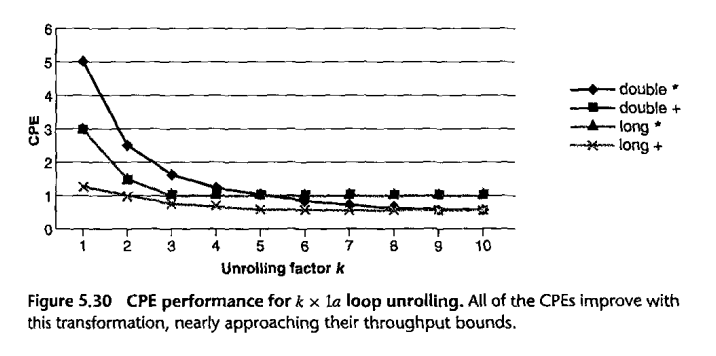# Week 10 notes

Comp 264-002, Spring 2019,MWF, 11:30-12:20, Cuneo 218

Chapter 3:

Section 3.1
Section 3.2
Section 3.3
Section 3.4
Section 3.5

Fixes to dir assignment (due to wrong file sizes); new due date

### Binary Bomb

Where is the secret phase?

What does initialize_bomb do?

Where, in general, is code loaded into memory? (See memory.c)

### Chapter 5:

combine1, BOH3 p 507

void combine1(vec_ptr v, data_t *result) {
*result = IDENT;
for (int i=0; i<vec_length(v); i++) {
data_t val;
get_vec_element(v, i, &val);
*result = *result OP val;
}
}

with OP=+, this takes ~22.7 clocks per loop cycle for integers with no optimization flag at all, and 10.12 with -O1 (BOH3 p 508). Floating point multiplication is almost the same: 20.2 with no optimization (faster!) and 11.14 with.

Next steps:

2. move vec_length() call out of loop: 7.02 clocks (p 513; combine2()), 11.03 with floating-point multiplication (again with -O1)

int length=vec_length(v);
for (int i=0; i < length; i++) {
data_t val;
get_vec_element(v, i, &val);
*result = *result OP val;
}

3. Eliminate the get_vec_element() call:

int length=vec_length(v);
data
_t *data = get_vec_start(v);
for (int i=0; i<length; i++) {
*dest = *dest OP data[i];
}

This turns out not to help at all. Accessors don't always come with a cost! (p 513, combine3(), 7.17/11.03)

4. move indirect memory reference out of loop: 1.27/5.04 clocks!! (p 515, combine4())

for (i=0; i<length; i++) acc = acc OP data[i];

Why can't the compiler optimizer figure out 2 and 4? For 4, the compiler can't verify that *dest isn't a location inside the array.

Four categories of registers within a tight loop:

• write-only
• local: values may be read or written, but the value at the end of the loop is not reused when the loop repeats
• loop: values are continuously read/written

Out-of-order processing and branch prediction / memory access

 BOH3 fig 5.12, p 523 int double latency issue capacity latency issue capacity addition 1 1 4 3 1 1 multiplication 3 1 1 5 1 2

That is, int addition takes 1 clock to perform; double multiplication takes 5 clocks. Issue time = min time between two consecutive operations, and capacity is the number of units capable of performing the operation.

Having issue < latency is due to pipelining: we can have 3 floating-point mults in process, in 3 different stages, for an average throughput of 1/clock. (The three stages are exponent processing, adding the fractional parts, and rounding)

Throughput is capacity/issue.

The fundamental bound on integer latency is 1 for +, 3 for *. For floating point these are 3 and 5.

The throughput bound is 0.5 and 1 for integer, 1 and 0.5 for float.

combine4 pretty much hits the latency bound for integer * and both float operation. Integer + is still slower. Here are the times for combine4(), and the corresponding latency and throughput bounds:

 int + int * double + double * combine4 1.27 3.01 3.01 5.01 latency bound 1.0 3.0 3.0 5.0 throughput bound 0.5 1.0 1.0 0.5

The throughput bound on floating-point * is double that of + because there are two multiplication units and only one adder. From fig 5.12, it would seem that the int + throughput should be 1/4, but there is a memory constraint: there are four adder units, but only two load-from-memory units.

strcmp loop example, BOH3 p 510

void lower1(char * s) {
for (int i=0; i<strlen(s); i++) {
if ('A' <= s[i] && s[i] <= 'Z') {
s[i] += 'a' - 'A';
}
}
}Why doesn't the optimizer fix this?

Data-flow analysis#### Loop unrolling

combine5:
for (i=0; i<length; i+=2) acc = (acc OP data[i]) OP data[i+1];

This is the 2x1 loop unrolling. There is also a 3x1 data unrolling, but 2x1 improves the clocks per element to 1.01, so there's not much point.

Actually we can do better. Here is the loop from combine6:

combine6:
for (i=0; i<length; i+=2) {
acc0 += data[i];
acc1 += data[i+1];
}

This is "2x2" unrolling: two operations per loop, two accumulators. Now the additions no longer need to be done sequentially! The CPEs fall to 0.81 / 1.51 / 1.51 / 2.51  (fig 5.21)

With kxk unrolling, we can approach the throughput bounds:Note that we are now doing the operations in a different order. This may make a difference to the result for floating-point operations. However, that usually happens only in peculiar circumstances.

combine7:

for (i=0; i<length; i+=2) acc = acc OP (data[i] OP data[i+1]);

What is the difference?

Combine7 times, though, are 1.01/1.51/1.51/2.51, versus combine5 times of 1.01/3.01/3.01/5.01. The improvement is the same as combine6, except for integer addition. What gives? It turns out the adder can be adding data[i] and data[i+1] at the same time the previous data sum is being added to acc.

If we apply kx1 unrolling using this technique, we get very close to the throughput bounds for integer addition with k~5, while for double multiplication we get close to the throughput bound around k~9. See the graph in BOH3 p 545.### Precomputed tables

Counting 1-bits: value of pre-computed tables.